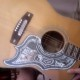### one step forward, two steps back: vlorbik’s seven-color theoremokay. not so much a theorem as a simple brute *fact*…
a perfectly *obvious* fact but one that i managed
to overlook for years. (this kind of thing
happens all the time of course.)

**Directions
let “clockwise” be the “positive” direction
(for this post only; the usual [trig class]
convention is to use the opposite orientation).
let “up” be “up” and let “down” be “down”.
let “day” and “night” be undefined.

**Seven-Color Space
seven-color space has seven colors.
as follows.

there are three Primaries: Red, Yellow, & Blue.
PC = {R, Y, B}.

there are three Secondaries: Green, Purple, and Orange.
SC = {G, P, O}
(of course O\not=0; this is obvious
as i type but milages vary: your
internet ain’t like mine).

there is one Ideal: Mud.
IC= {M}.

**Points
the set
PS = {R, Y, B, G, P, O, M}
is called the Underlying Set.
its elements are called “points”
of the space (or of U). of course,
its elements are *also* called “colors”.

**Lines
certain three-element subsets of U
are singled out and given the name “Lines”.
specifically, the lines are
{R, Y, O}, {R, B, P}, {Y, B, G}
(the “blends”… red and yellow paints
mix together to form orange, for example),
{R, G, M}, {Y, P, M}, {B,O, M}
(the “blurs”… pairs of “opposite” colors
of paint [like red and green] form a
Muddy neutral non-color), and
{G, P, O}
(the “secondaries”; we have encountered
this set before as SC).

for calculations, we will of course suppress
the set braces; we may conveniently denote
the set of lines for seven-color space as

LS = {RYO, RBP, YBG, RGM, YPM, BOM, GPO}.

(the understanding here is that the XYZ
stands for the *unordered* triple
{X, Y, Z} (= {X, Z, Y} = … = {Z, Y, X});
the *set* of colors and *not* their order
is what makes a line a line.)

The Standard Permutation
like *any* seven objects, the colors of CS
can be listed in any of 5040 (= 7*6*5*4*3*2*1)
orderings. it’s convenient to fix a *particular*
ordering from very early on in the discussion.
we have chosen
0: Mud
1: Red
2: Blue
3: Green
4: Purple
5: Yellow
6: Orange
as our Standard Permutation:
M_R_B_G_P_Y_O
(“mister big pie, oh!”).

The Regular Heptagon
(vlorbik’s seven-color theorem)

label the vertices of a regular heptagon
(clockwise) with the colors in the standard
permutation.

the circle-like nature of the heptagon
induces a “cyclic” structure on the colors,
which we can now think of as
M_R_B_G_P_Y_O_M_R_B_G_P_Y_O_M_R_B_G_P_Y_O…
“circling around forever”.

mark any vertex.
M_R_B_G_P_Y_*O*_M_R_B_G_P_Y_*O*_M_R_B_G_P_Y_*O*_…
(i have “marked the orange vertex”).
we can now compute one of the *lines*
[one that includes the “marked” color]
by going… this is “vlorbik’s theorem”…
Two Steps Forward and One Step Back
from the marked color:
“two steps forward” from O, for example,
gives us R (read to the right), and “one
step back” gives us Y; sure enough we get
one of the “blend”s: {R, Y, O} is one of
the Lines of seven-color space.

rotating the whole heptagon
(through an angle of 2\pi/7
or any integer multiple thereof)
permutes the colors in such a way
that lines are taken to lines.

only 168 of the 5040 permutations
of {R, B, Y, O, P, G, M}
share the property that “lines
are taken to lines”. i’ve just
drawn them all: announcing
Math Ed Zine #2. send money.

#### 1 Comment

1.vlorbik

https://vlorbik.wordpress.com/2014/03/14/the-poster-will-look-more-finished-the-zine-is-another-story/

at least one page is wrong.
eventually i’ll draw all 168 again
if universe spares me long enough…

• ## (Partial) Contents Page

Vlorbik On Math Ed ('07—'09)
(a good place to start!)